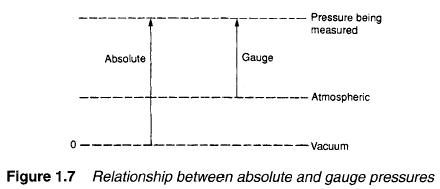﻿ Definition of terms, Pressure – Hydraulic Schematic Troubleshooting

## Definition of terms, Pressure

Pressure occurs in a fluid when it is subjected to a force. In Figure 1.4 a force F is applied to an enclosed fluid via a piston of area A. This results in a pressure P in the fluid. Obviously increasing the force increases the pressure in direct proportion. Less obviously, though, decreasing piston area also increases pressure. Pressure in the fluid can therefore be defined as the force acting per unit area, or: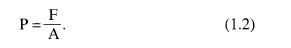Although expression 1.2 is very simple, there are many different units of pressure in common use. In the Imperial fps system, for example, F is given in lbs f and A is given in square inches to give pressure measured in pound force per square inch (psi).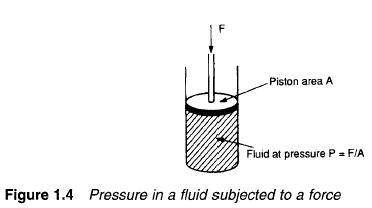In metric systems, F is usually given in kgf and A in square centimetres to give pressure in kilogram/force per square centimetre (kgf cm-2).

The SI system defines pressure as the force in newtons per square metre (N m-2). The SI unit of pressure is the pascal (with 1 Pa =1 N m-3). One pascal is a very low pressure for practical use, however, so the kilopascal (1 kPa=103pa) or the megapascal (1 MPa = 10 6 Pa) are more commonly used.

Pressure can also arise in a fluid from the weight of a fluid. This is usually known as the head pressure and depends on the height of fluid. In Figure 1.5 the pressure at the bottom of the fluid is directly proportional to height h.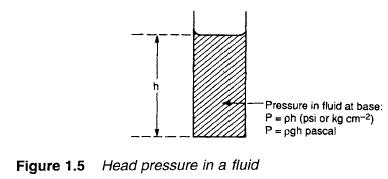In the Imperial and metric systems head pressure is given by: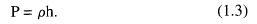where p is the density and h the height (both in the correct units) to give P in psi or kg cm -2.

In the SI system expression 1.3. is re-arranged as: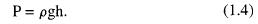where g is the acceleration due to gravity (9.81 ms -2) to give the pressure in pascal.

Pressure in a fluid can, however, be defined in terms of the equivalent head pressure. Common units are millimetres of mercury and centimetres, inches, feet or metres of water. The suffix wg (for water gauge) is often used when pressure is defined in terms of an equivalent head of water.

We live at the bottom of an ocean of air, and are consequently subject to a substantial pressure head from the weight of air above us. This pressure, some 15 psi, 1.05 kg f cm -2, or 101 kPa, is called an atmosphere, and is sometimes used as a unit of pressure.

It will be noted that 100 kPa is, for practical purposes, one atmosphere As this is a convenient unit for many applications 100 kPa (105 Pa or 0.1 MPa) has been given the name bar. Within the accuracy of instrumentation generally found in industry 1 bar = 1 atmosphere.

There are three distinct ways in which pressure is measured, shown in Figure 1.6. Almost all pressure transducers or transmitters measure the pressure difference between two input ports. This is known as differential pressure, and the pressure transmitter in Figure 1.6a indicates a pressure of P1-P2.

In Figure 1.6b the low pressure input port is open to atmosphere, so the pressure transmitter indicates pressure above atmospheric pressure. This is known as gauge pressure, and is usually denoted by a g suffix (e.g. psig). Gauge pressure measurement is almost universally used in hydraulic and pneumatic systems.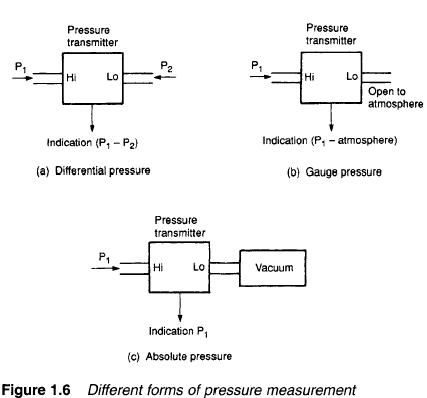Figure 1.6c shows the pressure transmitter measuring pressure with respect to a vacuum. This is known as absolute pressure and is of importance when the compression of gases is considered. The relationship between absolute and gauge pressure is illustrated in Figure 1.7. Pressure measurement and gas compression are discussed in later sections. Table 1.4 compares units of pressure. A typical hydraulic system operates at 150 bar, while typical pneumatic systems operate at 10 bar.